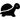## Overview

Figure and Ground

The distinction between figure and ground in art is compared to the distinction between theorems and nontheorems in formal systems. The question "Does a figure necessarily contain the same information as its ground?" leads to the distinction between recursively enumerable sets and recursive sets.

### Questions

1. People tend to believe intuitively, when they learn about prime numbers, that 1 is prime. According to the definitions used in number theory, however, 1 is neither prime nor composite. What ensures that 1 is not prime in the DND-system -- that is, that `P-` is not a theorem?
2. What would happen to the DND-system if `P-` were an axiom?
3. In the bottom-up decision procedure described on p. 48, why is it important that the axioms are ordered with the simplest (shortest) axiom first? What could go wrong if the axioms were put into the bucket in a different order?
4. Why is the FIGURE-FIGURE Figure shaped like that? If you think it's just an arbitrary shape that tiles the plane, reread p. 67.
5. Solve the puzzle on p. 73. What number comes next in the sequence 1, 3, 7, 12, 18, 26, 35, 45, 56, 69, ...? How is this like the FIGURE-FIGURE Figure?
6.Is the MIU-system recursive? Can you make a set of rules that characterize its negative space?

## Topics

### The terms "recursively enumerable" and "recursive"

You may find the use of the term "recursive" in this chapter confusing, because it has very little to do with the current popular meaning of "recursive" -- the one from computer science. The kind where when you Google "recursion" you get a link to the Google search for "recursion".

We'll see that kind of recursion in GEB too, but not yet.

Here, "recursively enumerable" just means that you can enumerate all the things in a certain set, and "recursive" means that you can also enumerate the things that aren't in the set, and therefore decide whether something is in the set or not.

If you want to update the terminology to what computer scientists now use, then replace this sense of "recursive" with "decidable".

There's also "re-cursive", which is just a pun Hofstadter introduces as he transfers this idea into the world of visual art.

### Typographical systems

Pretty much all formal systems in this book will be defined using typographical rules. So far, the examples you've seen are the MIU-system, the pq-system, and now the tq-system and its variants. "Typographical" is a term pretty much used only by Hofstadter in this sense, but computer scientists should recognize the concept.

The key features of typographical operations are

• They are simple enough that they resemble the fundamental steps of an algorithm. You can think of every step as requiring a constant amount of work. You can't do something hard like factor a large number in one typographical step.
• They are general enough that you can make any algorithm out of them. Typographical rules, if you get to choose what the rules are, are Turing-complete.
• Because the intermediate states are strings of symbols, those states are easy to show in a book.

A programming language that qualifies as a typographical system is ➟ M4 (computer language). This language is dated, but it's still in active use, deep in the internals of UNIX programs that few would consider messing with the internals of.

Unlike most programming languages, though, typographical systems can be non-deterministic. Programming languages tend to have one state lead directly to one other state, but from `MII` you can produce `MIIII` or `MIIU`, and no rule forces you to choose one -- you can do either one in a single step.

### Figure and ground in music

A quote by Claude Debussy: "Music is the space between the notes."

Debussy is probably being hyperbolic as a way to get people to think about their performance, but can you think of a piece of music that actually has an identifiable "figure" in the space between the notes?

## Commentary

(This section is for adding your thoughts about the chapter. Sign what you write with your user name. Others may edit this section for length later. More free-form, unedited discussion can take place in the comment section below.)